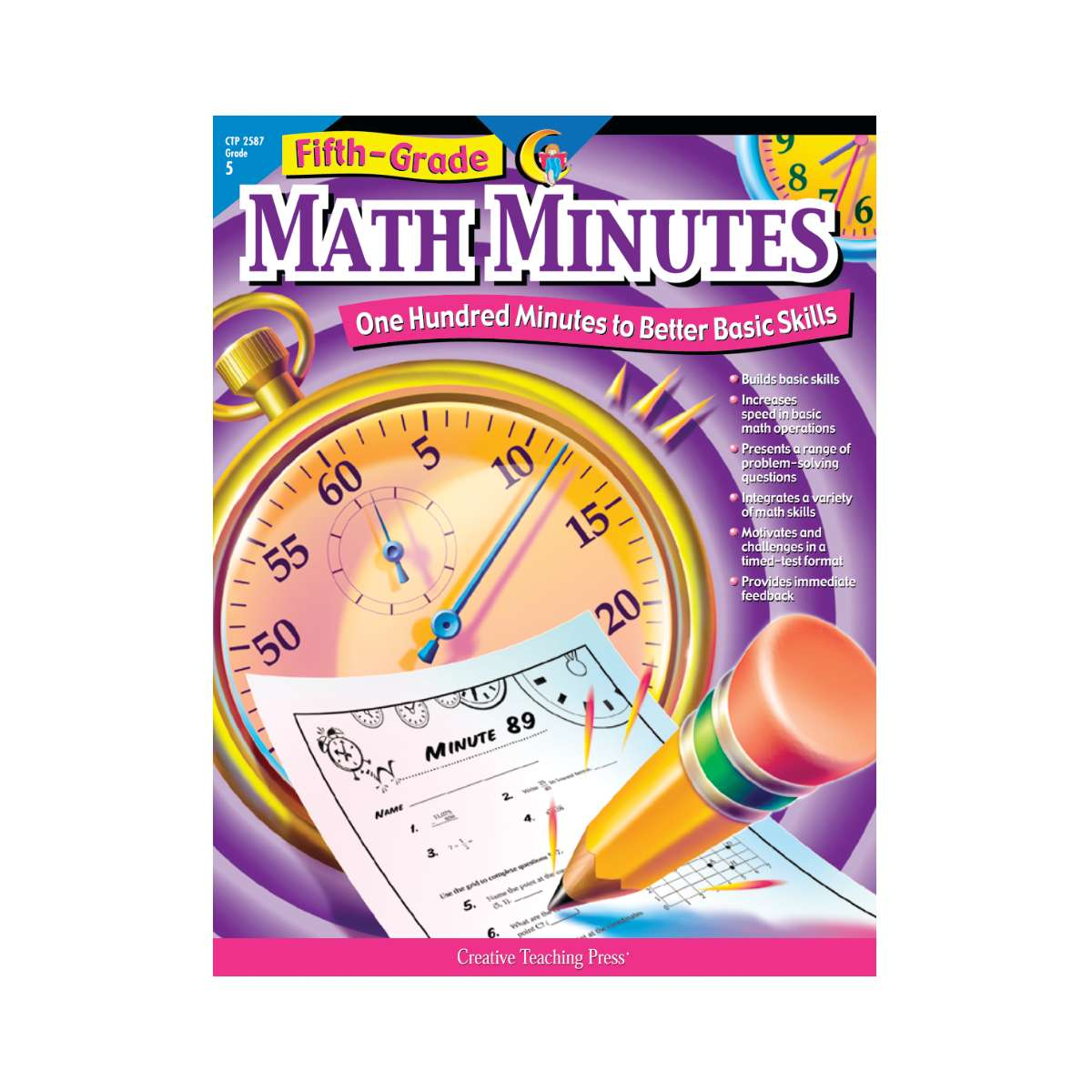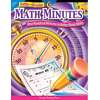•Math Minutes, Grade 5

Product Number: CTP2587
Now there's a way for students to practice their math skills in a timed situation, enhance their overall math fluency, and ha...
Quantity

Now there's a way for students to practice their math skills in a timed situation, enhance their overall math fluency, and have fun at the same time! Fifth-Grade Math Minutes provides students with practice in every key area of fifth-grade math instruction, including:

• multiplication
• division
• divisibility
• time
• graphs
• fact families
• solid figures
• ratio/percent
• area
• tables
• geometry
• volume
• mixed numbers
• multiplying fractions
• prime numbers
• standard measurement
• metric measurement
• and more!

Fifth-Grade Math Minutes features 100 "Minutes." Each Minute consists of ten classroom-tested problems of varying degrees of difficulty, integrating problem solving and basic math skills. This unique format offers students an ongoing opportunity to improve their own fluency in a manageable, nonthreatening format. The quick, one-minute format combined with instant feedback makes this challenging and motivational assignment students will look forward to each day. Students become active learners as they discover mathematical relationships and apply acquired understanding to complex situations and to the solution of realistic problems in each Minute.

CTP2587